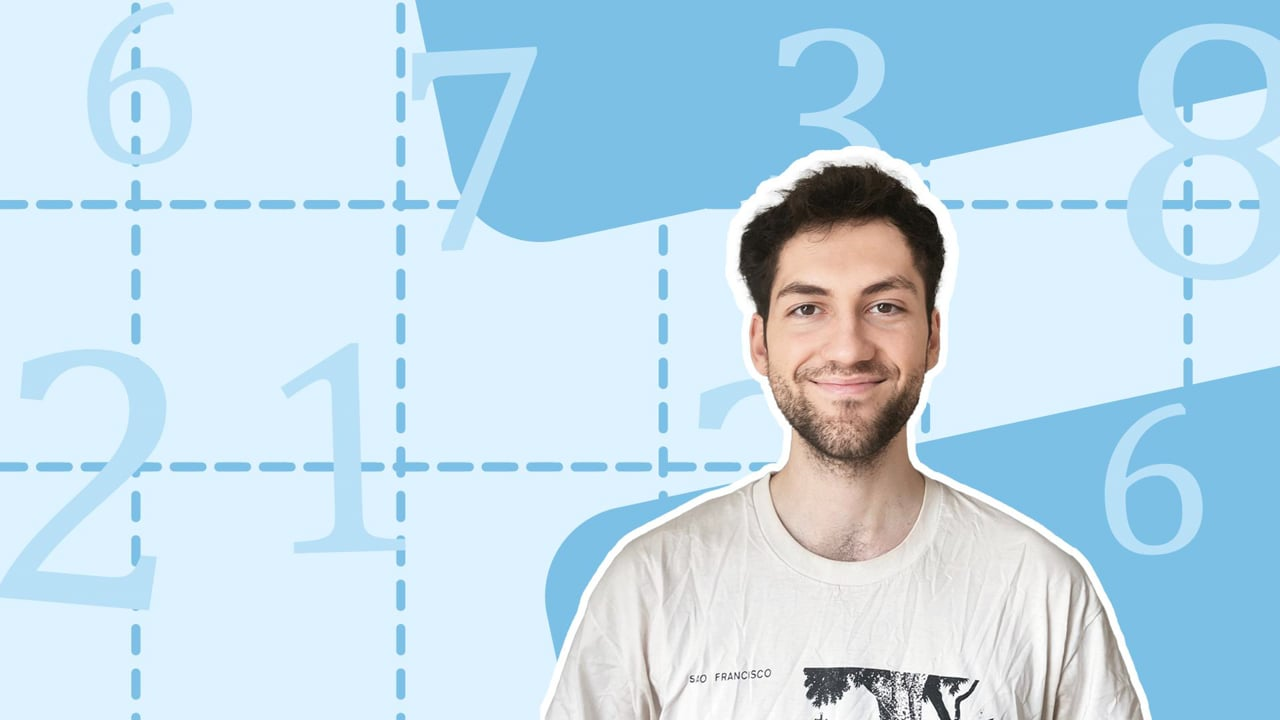Chapter OverviewMaths

Number and place value

Multiplication and division

Fractions

Measurement

Geometry - properties of shapes

Geometry - position and direction

Statistics

Ratio and proportion

Algebra

Maths

# Inverse operations to check calculations0%

Summary

# Inverse operations to check calculations

## ​​In a nutshell

Addition and subtraction are inverse operations to each other. By re-expressing a calculation, you can check your answer with the inverse operation.

## Inverse operation

To be an inverse operation means to undo what the operation did. If you add five, this can always be undone by subtracting five. Hence the inverse operation of addition is subtraction. Similarly, if you subtract two, you can always undo this by adding two. Therefore addition is the inverse operation of subtraction.

##### Example 1

Some number plus six equals fourteen. What is the number?

First express this as a mathematical equation. Since you don't yet know the number, call it $x$. So:

$x+6=14$

To find $x$, find the inverse of what has been applied to $x$, and apply it to $14$. The inverse of adding $6$ is subtracting $6$. Hence the following is also true:

$x=14-6$​​

This gives $8$, so

$\underline{x=8}$.

Beyond being used to solve problems like the example above, inverse operations are helpful when checking answers.

##### Example 2

Calculate $74-49$.

$\begin {array} { c c c}& ^6\cancel7 & ^14 \\- & 4 & 9 \\ \hline& 2& 5\\ \hline\end {array}$​​

So $74-49=25$. Now you can check this is correct using the inverse operation. Initially, you subtracted $49$ from $74$ to find $25$. The inverse is to add $49$ to $25$. You can now check if this does give $74$:

$\begin {array} { c c c}& \overset{1}2 & 5 \\+ & 4 & 9 \\ \hline& 7 & 4 \\ \hline\end {array}$​​

As expected, you get $74$, so you can be confident that $74-49=25$.

##### Example 3

Calculate $119+15$.​​

$\begin{array}{cccccccccccccc}&&&+1&&+1&&+1&&+1&&+1&&& \\& &&\curvearrowright &&\curvearrowright &&\curvearrowright&&\curvearrowright &&\curvearrowright &&\\118 &&\underline{119}&&120&&121&&122&&123&&\underline{124}&& \\|&&|&&|&&|&&|&&|&&| \\ \hline\end{array}$​​

Now count up the one ten:

$\begin{array}{cccccccccccccc}&&&&&&&+10&&&&& \\& &&&& &&\curvearrowright&& &&&&\\94&&104&&114&&\underline{124}&&\underline{134}&&144&&154&& \\|&&|&&|&&|&&|&&|&&| \\ \hline\end{array}$​​

To check this answer, find the inverse of adding $15$, and apply this inverse to $134$. The inverse is subtracting $15$. Start with the five units:

$\begin{array}{cccccccccccccc}&&&-1&&-1&&-1&&-1&&-1&&& \\& &&\curvearrowleft &&\curvearrowleft &&\curvearrowleft&&\curvearrowleft &&\curvearrowleft &&\\128 &&\underline{129}&&130&&131&&132&&133&&\underline{134}&& \\|&&|&&|&&|&&|&&|&&| \\ \hline\end{array}$​​

Then subtract the one ten:

$\begin{array}{cccccccccccccc}&&&&&&&-10&&&&& \\& &&&& &&\curvearrowleft&& &&&&\\89&&99&&109&&\underline{119}&&\underline{129}&&139&&149&& \\|&&|&&|&&|&&|&&|&&| \\ \hline\end{array}$​​

See that by inverting the process, you have returned to $119$, hence you can be more confident that $119+15=\underline{134}$.

Note: You need not stick to just the column method, or just the number line method. Use whichever you prefer, unless it is stated to use one particular method.